# 单目相机成像过程

Qt自定义控件之仪表盘3–雷达扫描图

# 单目相机成像过程

## 01 理想情况下相机成像模型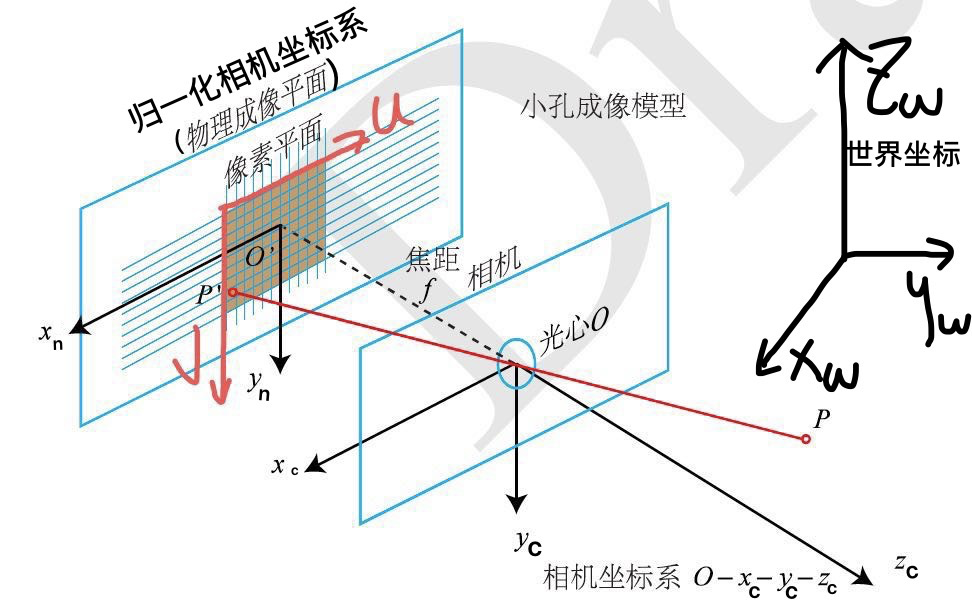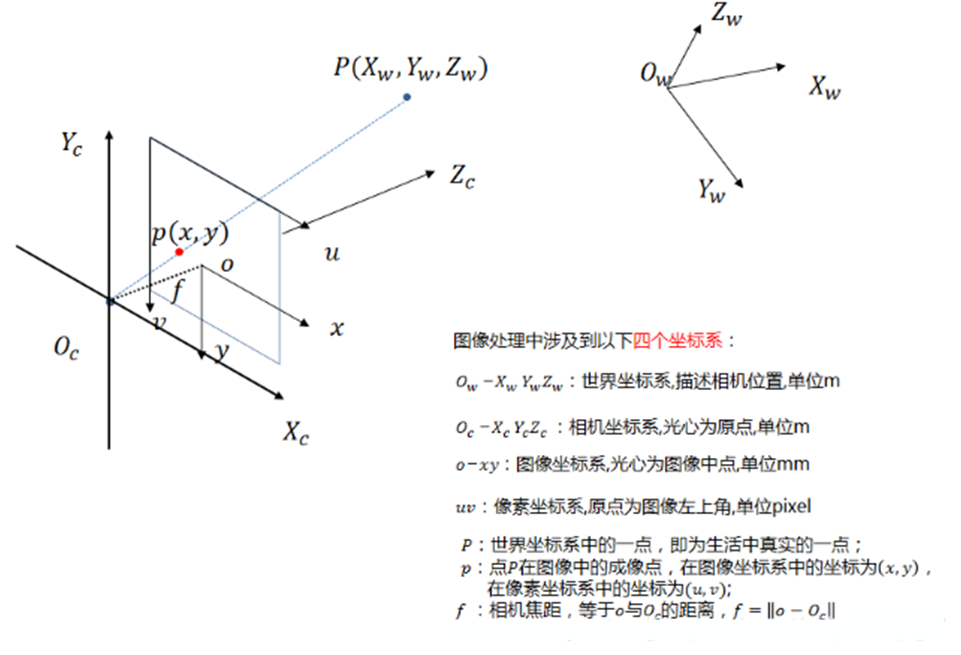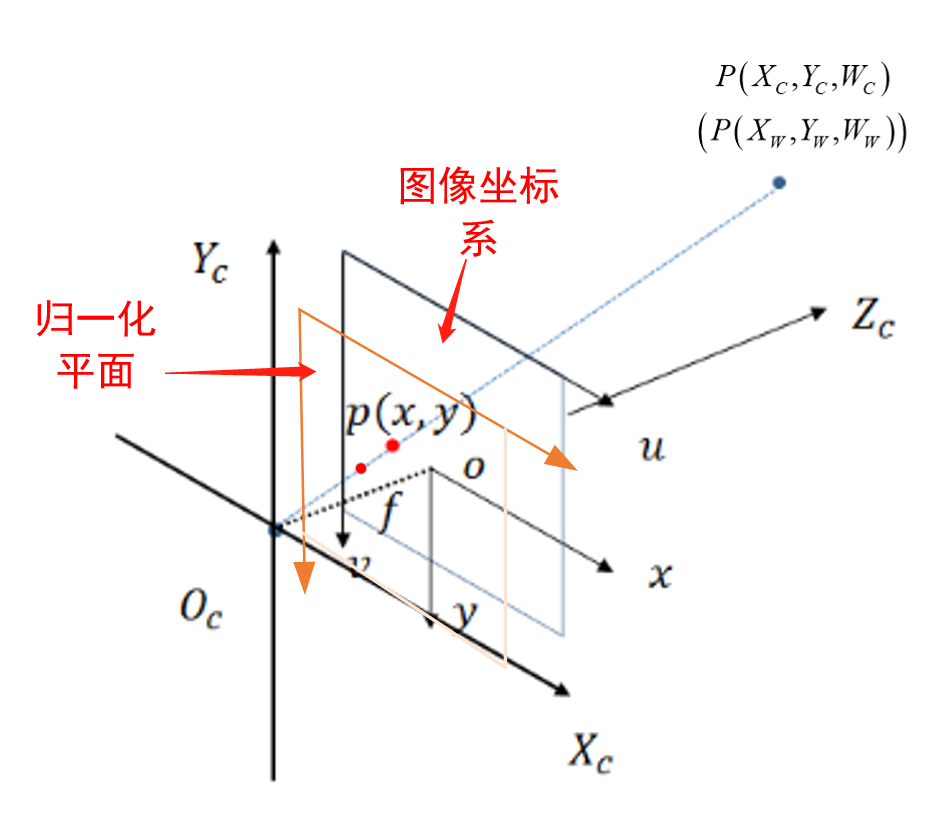### 1）世界坐标系 -> 相机坐标系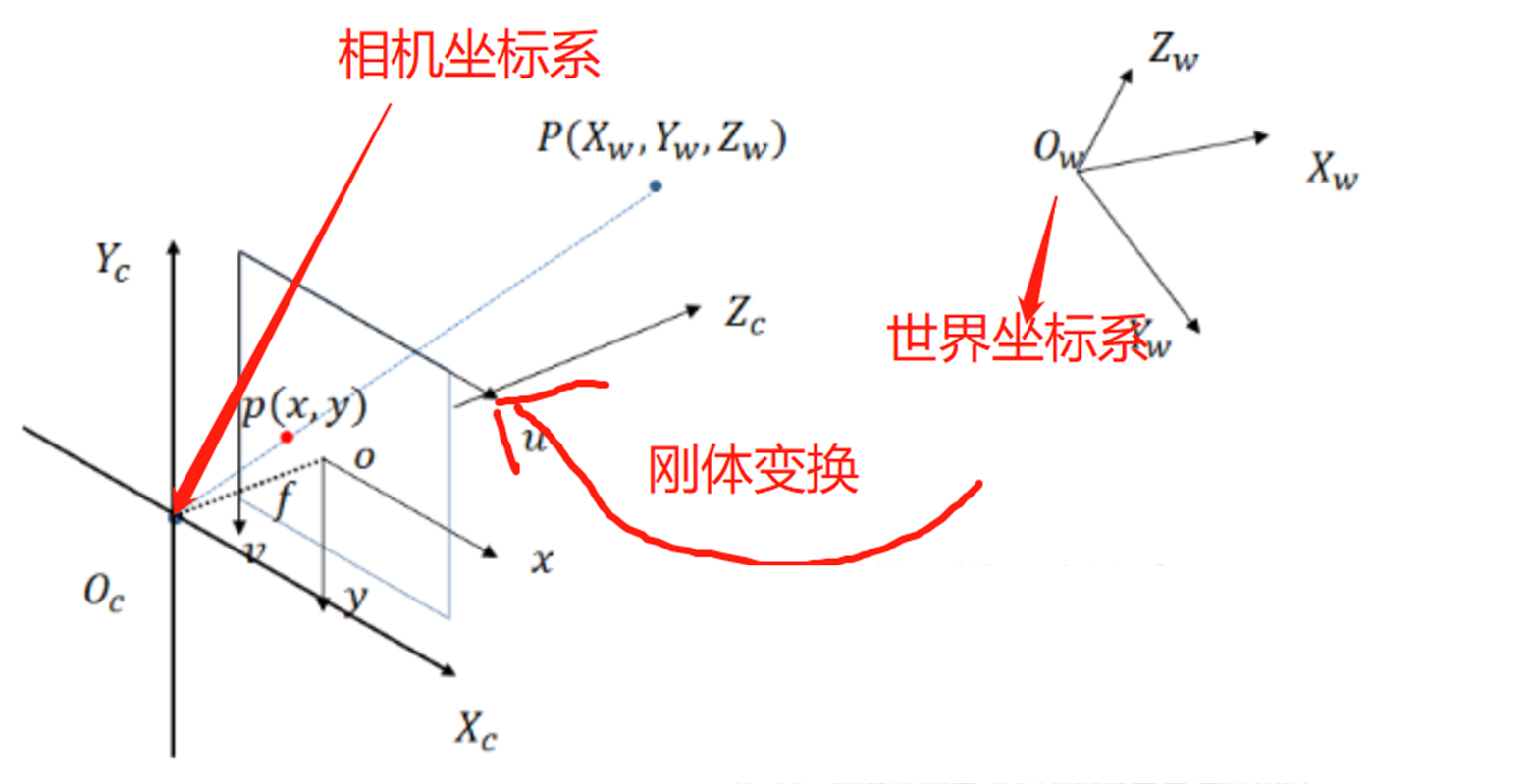$\left[ {\begin{array}{*{20}{c}} {{X_c}}\\ {{Y_c}}\\ {{Z_c}} \end{array}} \right] = \left[ {\begin{array}{*{20}{c}} {{r_{11}}}&{{r_{12}}}&{{r_{13}}}\\ {{r_{21}}}&{{r_{22}}}&{{r_{23}}}\\ {{r_{31}}}&{{r_{32}}}&{{r_{33}}} \end{array}} \right]\left[ {\begin{array}{*{20}{c}} {{X_W}}\\ {{Y_W}}\\ {{Z_W}} \end{array}} \right] + \left[ {\begin{array}{*{20}{c}} {{T_x}}\\ {{T_y}}\\ {{T_z}} \end{array}} \right]$

$\underbrace {\left[ {\begin{array}{*{20}{c}} {{X_C}}\\ {{Y_C}}\\ {{Z_C}}\\ 1 \end{array}} \right]}_{相机坐标系} = \underbrace {\left[ {\begin{array}{*{20}{c}} {{R_{3 \times 3}}}&{{T_{3 \times 1}}}\\ 0&1 \end{array}} \right]}_{刚体变换}\underbrace {\left[ {\begin{array}{*{20}{c}} {{X_W}}\\ {{Y_W}}\\ {{Z_W}}\\ 1 \end{array}} \right]}_{世界坐标系}$

### 2）相机坐标系 -> 图像坐标系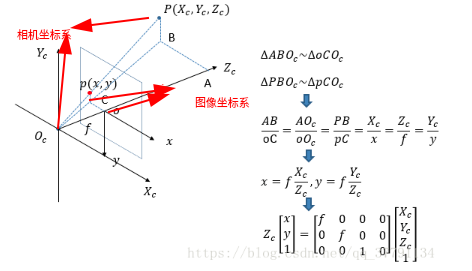${Z_c}\underbrace {\left[ {\begin{array}{*{20}{c}} x\\ y\\ 1 \end{array}} \right]}_{\rm{图像坐标系}} = \underbrace {\left[ {\begin{array}{*{20}{c}} f&0&0&0\\ 0&f&0&0\\ 0&0&1&0 \end{array}} \right]}_{相似三角}\underbrace {\left[ {\begin{array}{*{20}{c}} {{X_C}}\\ {{Y_C}}\\ {{Z_C}}\\ 1 \end{array}} \right]}_{相机坐标系}$

### 3）图像坐标系 -> 像素坐标系

• 坐标原点不同：图像坐标系，成像平面的中心；像素坐标系，成像平面左上角
• 单位不同：图像坐标系，单位mm，属于物理单位；像素坐标系，单位pixel（$$1 \ pixel= dx \ or \ dy \ mm$$），平常描述一个像素点都是几行几列；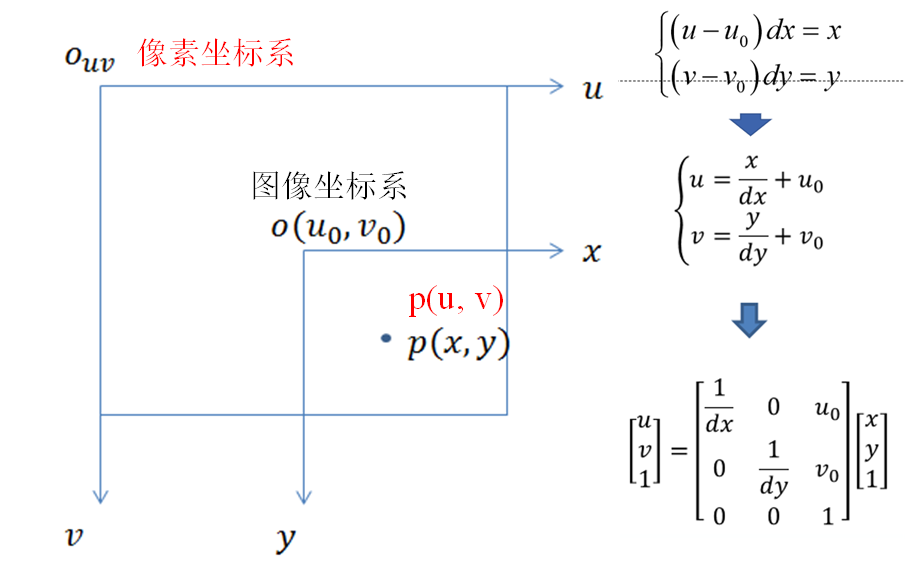$\underbrace {\left[ {\begin{array}{*{20}{c}} u\\ v\\ 1 \end{array}} \right]}_{像素坐标系} = \underbrace {\left[ {\begin{array}{*{20}{c}} {\frac{1}{{dx}}}&0&{{u_0}}\\ 0&{\frac{1}{{dy}}}&{{v_0}}\\ 0&0&1 \end{array}} \right]}_{平移+缩放}\underbrace {\left[ {\begin{array}{*{20}{c}} x\\ y\\ 1 \end{array}} \right]}_{图像坐标系}$

### 4）总结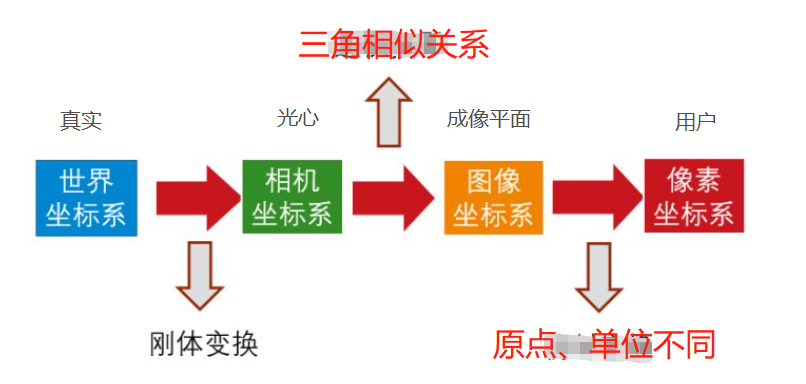1. 世界坐标系到相机坐标系

$\underbrace {\left[ {\begin{array}{*{20}{c}} {{X_C}}\\ {{Y_C}}\\ {{Z_C}}\\ 1 \end{array}} \right]}_{相机坐标系} = \underbrace {\left[ {\begin{array}{*{20}{c}} {{R_{3 \times 3}}}&{{T_{3 \times 1}}}\\ 0&1 \end{array}} \right]}_{刚体变换}\underbrace {\left[ {\begin{array}{*{20}{c}} {{X_W}}\\ {{Y_W}}\\ {{Z_W}}\\ 1 \end{array}} \right]}_{世界坐标系}$

2. 相机坐标系到图像坐标系

${Z_c}\underbrace {\left[ {\begin{array}{*{20}{c}} x\\ y\\ 1 \end{array}} \right]}_{\rm{图像坐标系}} = \underbrace {\left[ {\begin{array}{*{20}{c}} f&0&0&0\\ 0&f&0&0\\ 0&0&1&0 \end{array}} \right]}_{相似三角}\underbrace {\left[ {\begin{array}{*{20}{c}} {{X_C}}\\ {{Y_C}}\\ {{Z_C}}\\ 1 \end{array}} \right]}_{相机坐标系}$

3. 图像坐标系到像素坐标系

$\underbrace {\left[ {\begin{array}{*{20}{c}} u\\ v\\ 1 \end{array}} \right]}_{像素坐标系} = \underbrace {\left[ {\begin{array}{*{20}{c}} {\frac{1}{{dx}}}&0&{{u_0}}\\ 0&{\frac{1}{{dy}}}&{{v_0}}\\ 0&0&1 \end{array}} \right]}_{平移+缩放}\underbrace {\left[ {\begin{array}{*{20}{c}} x\\ y\\ 1 \end{array}} \right]}_{图像坐标系}$

${Z_c}\underbrace {\left[ {\begin{array}{*{20}{c}} u\\ v\\ 1 \end{array}} \right]}_{像素坐标系} = \underbrace {\left[ {\begin{array}{*{20}{c}} {\frac{1}{{dx}}}&0&{{u_0}}\\ 0&{\frac{1}{{dy}}}&{{v_0}}\\ 0&0&1 \end{array}} \right]}_{03 \ 平移+缩放}\underbrace {\left[ {\begin{array}{*{20}{c}} f&0&0&0\\ 0&f&0&0\\ 0&0&1&0 \end{array}} \right]}_{02\ 相似三角形}\underbrace {\left[ {\begin{array}{*{20}{c}} {{R_{3 \times 3}}}&{{T_{3 \times 1}}}\\ 0&1 \end{array}} \right]}_{01 \ 刚体变换}\underbrace {\left[ {\begin{array}{*{20}{c}} {{X_W}}\\ {{Y_W}}\\ {{Z_W}}\\ 1 \end{array}} \right]}_{世界坐标系}$

${Z_c}\underbrace {\left[ {\begin{array}{*{20}{c}} u \\ v \\ 1 \end{array}} \right]}_{像素坐标系} = \underbrace {\left[ {\begin{array}{*{20}{c}} {{f_x}}&0&{{u_0}} \\ 0&{{f_y}}&{{v_0}} \\ 0&0&1 \end{array}} \right]}_{M1：内参}\underbrace {\left[ {\begin{array}{*{20}{c}} {{R_{3 \times 3}}}&{{T_{3 \times 1}}} \end{array}} \right]}_{M2：外参}\underbrace {\left[ {\begin{array}{*{20}{c}} {{X_W}} \\ {{Y_W}} \\ {{Z_W}} \\ 1 \end{array}} \right]}_{世界坐标系}$

## 02 考虑畸变情况下相机成像模型

### 1）径向畸变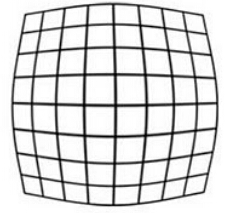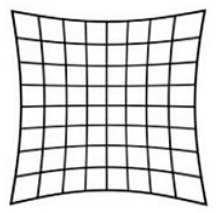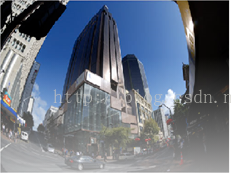$\left[ {\begin{array}{*{20}{c}} {x’}\\ {y’} \end{array}} \right] = (1 + {k_1}{r^2} + {k_2}{r^4} + {k_3}{r^6})\left[ {\begin{array}{*{20}{c}} x\\ y \end{array}} \right]$

• $$r$$ 为曲率半径，有：$$r^2 =x^2 + y^2$$
• $$k_1,k_2,k_3$$ 为径向畸变系数；
• $$x, y$$ 为发生畸变后角点的坐标，也就是我们实际看到的；
• $$x’,y’$$ 为畸变矫正，也就是去除畸变后的正确坐标；

### 2）切向畸变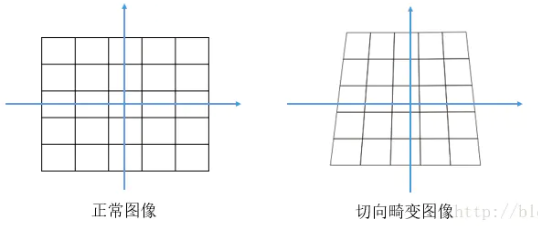$\left[ {\begin{array}{*{20}{c}} x’\\ y’ \end{array}} \right] = \left[ {\begin{array}{*{20}{c}} {2{p_1}xy + {p_2}\left( {{r^2} + 2{x^2}} \right)}\\ {2{p_2}xy + {p_1}\left( {{r^2} + 2{y^2}} \right)} \end{array}} \right]$

### 3）合并考虑畸变

$\left[ {\begin{array}{*{20}{c}} {x’}\\ {y’} \end{array}} \right] = \underbrace {\left( {1 + {k_1}{r^2} + {k_2}{r^4} + {k_3}{r^6}} \right)\left[ {\begin{array}{*{20}{c}} x\\ y \end{array}} \right]}_{径向畸变} + \underbrace {\left[ {\begin{array}{*{20}{c}} {2{p_1}xy + {p_2}\left( {{r^2} + 2{x^2}} \right)}\\ {2{p_2}xy + p1\left( {{r^2} + 2{y^2}} \right)} \end{array}} \right]}_{切向畸变}$

• $$k_1,k_2,k_3$$ 为径向畸变系数；
• $$p_1,p_2$$ 为切向畸变系数；

• 世界坐标系 -> 相机坐标系，刚体变换，不存在畸变现象；
• 相机坐标系 -> 图像坐标系，也就是成像过程，理想情况下是相似三角形，但实际由于相机制造、装配的原因，成像过程存在畸变现象；
• 图像坐标系 -> 像素坐标系，坐标原点、单位不同，仅仅平移与缩放，不存在畸变现象；

## 03 成像过程总结

• 某点世界坐标系为$$P(X_W,Y_W,Z_W)$$
• 对应的实际得到的像素坐标系为 $$P(u,v)$$（未矫正的）；
• 正确的像素坐标为 $$P(u’,v’)$$
• 假设我们已知畸变系数 $$k_1,k_2,k_3,p_1,p_2$$

1. 像素坐标系 -> 归一化坐标系

这个变换仅仅是平移与缩放，不存在畸变，因而只需要一个逆变换，归一化坐标 $$P=(x,y)^T$$ 推导如下：

$\begin{array}{c} \underbrace {\left[ {\begin{array}{*{20}{c}} u\\ v\\ 1 \end{array}} \right]}_{像素坐标} = \underbrace {\left[ {\begin{array}{*{20}{c}} {\frac{1}{{dx}}}&0&{{u_0}}\\ 0&{\frac{1}{{dy}}}&{{v_0}}\\ 0&0&1 \end{array}} \right]}_{平移+缩放}\underbrace {\left( {\underbrace {\left[ {\begin{array}{*{20}{c}} x\\ y\\ {1/f} \end{array}} \right]}_{归一化坐标} \times f} \right)}_{图像坐标} \\ \Downarrow \\ \underbrace {\left[ {\begin{array}{*{20}{c}} x\\ y\\ {1/f} \end{array}} \right]}_{归一化坐标} = \underbrace {\left( {{{\left[ {\begin{array}{*{20}{c}} {\frac{1}{{dx}}}&0&{{u_0}}\\ 0&{\frac{1}{{dy}}}&{{v_0}}\\ 0&0&1 \end{array}} \right]}^{ – 1}}\underbrace {\left[ {\begin{array}{*{20}{c}} u\\ v\\ 1 \end{array}} \right]}_{像素坐标}} \right)}_{图像坐标}/f \end{array}$

2. 归一化坐标系（带畸变的） -> 归一化坐标系（畸变矫正后）

在前一成像过程，也就是相机坐标系到归一化平面透射中，相机发生了畸变，因而我们需要将实际的归一化坐标 $$P=(x,y)^T$$ 纠正到理想的无畸变归一化坐标 $$P=(x’,y’)^T$$

$\left[ {\begin{array}{*{20}{c}} {x’}\\ {y’}\\ {1/f} \end{array}} \right] = \left[ {\begin{array}{*{20}{c}} {\left( {1 + {k_1}{r^2} + {k_2}{r^4} + {k_3}{r^6}} \right)x + 2{p_1}xy + {p_2}\left( {{r^2} + 2{x^2}} \right)}\\ {\left( {1 + {k_1}{r^2} + {k_2}{r^4} + {k_3}{r^6}} \right)y + 2{p_2}xy + {p_1}\left( {{r^2} + 2{y^2}} \right)}\\ {1/f} \end{array}} \right]$

3. 归一化坐标系（理想）-> 相机坐标系

理想的无畸变归一化坐标 $$P=(x’,y’)$$ 到相机坐标系，它们是相似三角形关系：

${Z_c}\underbrace {\left( {\underbrace {\left[ {\begin{array}{*{20}{c}} {x’}\\ {y’}\\ 1/f \end{array}} \right]}_{归一化坐标系（准确）} \cdot f} \right)}_{图像坐标} = \underbrace {\left[ {\begin{array}{*{20}{c}} f&0&0&0\\ 0&f&0&0\\ 0&0&1&0 \end{array}} \right]}_{相似三角形}\underbrace {\left[ {\begin{array}{*{20}{c}} {{X_C}}\\ {{Y_C}}\\ {{Z_C}}\\ 1 \end{array}} \right]}_{相机坐标} \\ \Downarrow \\ \left[ {\begin{array}{*{20}{c}} {{X_c}}\\ {{Y_c}}\\ {{Z_c}}\\ 1 \end{array}} \right] = f \cdot {Z_c} \cdot {\left[ {\begin{array}{*{20}{c}} f&0&0&0\\ 0&f&0&0\\ 0&0&1&0 \end{array}} \right]^{ – 1}}\left[ {\begin{array}{*{20}{c}} {x’}\\ {y’}\\ {1/f} \end{array}} \right]$

注：这里 $$3 \times 4$$ 矩阵的逆是伪逆。

4. 相机坐标系 -> 世界坐标系

相机坐标系到世界坐标系，仅仅是之前刚体变换的反变换：

$\underbrace {\left[ {\begin{array}{*{20}{c}} {{X_C}}\\ {{Y_C}}\\ {{Z_C}}\\ 1 \end{array}} \right]}_{相机坐标系} = \underbrace {\left[ {\begin{array}{*{20}{c}} {{R_{3 \times 3}}}&{{T_{3 \times 1}}}\\ 0&1 \end{array}} \right]}_{刚体变换}\underbrace {\left[ {\begin{array}{*{20}{c}} {{X_W}}\\ {{Y_W}}\\ {{Z_W}}\\ 1 \end{array}} \right]}_{世界坐标系} \\ \Downarrow \\ \left[ {\begin{array}{*{20}{c}} {{X_W}}\\ {{Y_W}}\\ {{Z_W}}\\ 1 \end{array}} \right] = {\left[ {\begin{array}{*{20}{c}} {{R_{3 \times 3}}}&{{T_{3 \times 1}}}\\ 0&1 \end{array}} \right]^{ – 1}}\left[ {\begin{array}{*{20}{c}} {{X_C}}\\ {{Y_c}}\\ {{Z_c}}\\ 1 \end{array}} \right]$

## 04 思考问题

### 1）问题一

$\left[ {\begin{array}{*{20}{c}} {x’}\\ {y’} \end{array}} \right] = \underbrace {\left( {1 + {k_1}{r^2} + {k_2}{r^4} + {k_3}{r^6}} \right)\left[ {\begin{array}{*{20}{c}} x\\ y \end{array}} \right]}_{径向畸变} + \underbrace {\left[ {\begin{array}{*{20}{c}} {2{p_1}xy + {p_2}\left( {{r^2} + 2{x^2}} \right)}\\ {2{p_2}xy + p1\left( {{r^2} + 2{y^2}} \right)} \end{array}} \right]}_{切向畸变}$

$s\tilde m = A\left[ {\begin{array}{*{20}{c}} {{R_{3 \times 3}}}&{{T_{3 \times 1}}} \end{array}} \right]\tilde M$

• $$s$$ 称为尺度因子；
• $\tilde m$ 为像素坐标系，$\tilde M$ 为世界坐标系；
• $$A$$ 为单应性矩阵；
• $$[R_{3 \times 3} \ T_{3\times1}]$$ 是外参矩阵；

### 2）问题二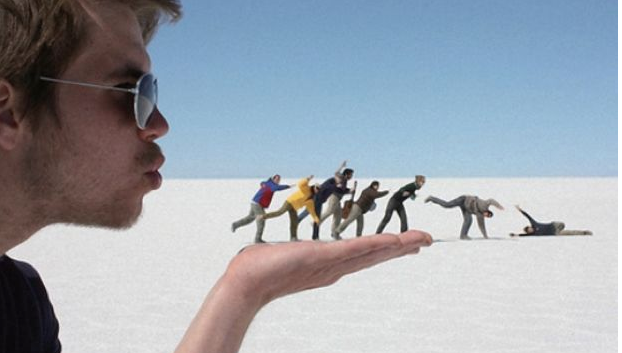Python接口自动化测试框架(Pytest+Allure+jsonpath+xlrd+excel、支持Restful接口规范)